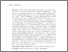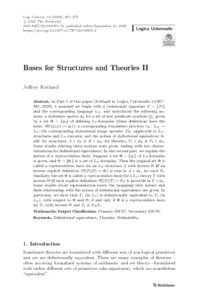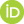# Bases for Structures and Theories II

Ketland, Jeffrey (2020) Bases for Structures and Theories II. Logica Universalis, 14. pp. 461-479.Preview
Text
Ketland2020_Article_BasesForStructuresAndTheoriesII.pdf

## Abstract

In Part I of this paper, I assumed we begin with a (relational) signature $P = \{P_i\}$ and the corresponding language $L_P$, and introduced the following notions: a \emph{definition system} $d_{\Phi}$ for a set of new predicate symbols $Q_i$, given by a set $\Phi = \{\phi_i\}$ of defining $L_P$-formulas (these definitions have the form: $\forall \overline{x}(Q_i(x) \iff \phi_i)$); a corresponding \emph{translation function} $\tau_{\Phi}: L_Q \to L_P$; the corresponding \emph{definitional image operator} $D_{\Phi}$, applicable to $L_P$-structures and $L_P$-theories; and the notion of \emph{definitional equivalence} itself: for structures $A + d_{\Phi} \equiv B + d_{\Theta}$; for theories, $T_1 + d_{\Phi} \equiv T_2 + d_{\Theta}$. Some results relating these notions were given, ending with two characterizations for definitional equivalence.

In this second part, we explain the notion of a \emph{representation basis}. Suppose a set $\Phi = \{\phi_i\}$ of $L_P$-formulas is given, and $\Theta = \{\theta_i\}$ is a set of $L_Q$-formulas. Then the original set $\Phi$ is called a \emph{representation basis} for an $L_P$-structure $A$ with inverse $\Theta$ iff an inverse explicit definition $\forall \x(P_i(\overline{x}) \iff \theta_i)$ is true in $A + d_{\Phi}$, for each $P_i$. Similarly, the set $\Phi$ is called a \emph{representation basis} for a $L_P$-theory $T$ with inverse $\Theta$ iff each explicit definition $\forall \overline{x}(P_i(\overline{x}) \iff \theta_i)$ is provable in $T + d_{\Phi}$. Some results about representation bases, the mappings they induce and their relationship with the notion of definitional equivalence are given. In particular, we show that $T_1$ (in $L_P$) is definitionally equivalent to $T_2$ (in $L_Q$), with respect to $\Phi$ and $\Theta$, if and only if $\Phi$ is a \emph{representation basis} for $T_1$ with inverse $\Theta$ and $T_2 \equiv D_{\Phi}T_1$.

 Export/Citation: EndNote | BibTeX | Dublin Core | ASCII/Text Citation (Chicago) | HTML Citation | OpenURL
 Social Networking:

Item Type: Published Article or Volume
Creators:
CreatorsEmailORCIDKetland, Jeffreyjeffreyketland@gmail.com0000-0002-5128-4387
Keywords: definitional equivalence; theoretical equivalence
Subjects: General Issues > Structure of Theories
Depositing User: Dr Jeffrey Ketland
Date Deposited: 29 Nov 2020 19:57
Item ID: 18459
Journal or Publication Title: Logica Universalis
Publisher: Springer
DOI or Unique Handle: https://doi.org/10.1007/s11787-020-00261-2
Subjects: General Issues > Structure of Theories
Date: 2020
Page Range: pp. 461-479
Volume: 14
URI: http://philsci-archive.pitt.edu/id/eprint/18459

### Monthly Views for the past 3 yearsView Item